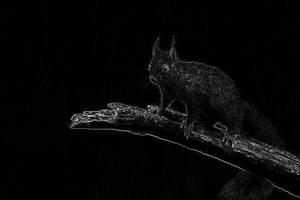# Python OpenCV – Roberts Edge Detection

• Last Updated : 19 Dec, 2021

Edge detection refers to a set of mathematical techniques for recognizing points in a digital image where the image brightness abruptly changes or, more formally, where there are discontinuities. The sharp fluctuations in image brightness are usually grouped into a collection of curved line segments called edges.

## Roberts Edge Detection

The Roberts operator measures a 2-D spatial gradient on an image in a straightforward, quick-to-compute manner. As a result, strong spatial gradient zones, which frequently correspond to edges, are highlighted. The operator’s input and output are both grayscale images in their most typical configuration. The estimated absolute magnitude of the input image’s spatial gradient at that position is represented by pixel values at each place in the output.

## Roberts cross operator

The Roberts cross operator is used in image processing and computer vision for edge detection. Lawrence Roberts proposed it in 1963, and it was one of the first edge detectors. The Roberts cross operator is a differential operator that approximates an image’s gradient via discrete differentiation, which is accomplished by computing the sum of the squares of the differences between diagonally adjacent pixels.

Approach:

The operator is made up of a pair of 2×2 convolution masks, as shown. One mask is merely the other turned 90 degrees. This is a lot like the Sobel operator.

These masks, one for each of the two perpendicular orientations, are designed to respond maximally to edges running at 45° to the pixel grid. The masks can be applied to the input image independently to produce separate gradient component measurements in each orientation (call theseand). These can then be combined to determine the absolute magnitude and orientation of the gradient at each site. The gradient magnitude is given byThe angle of orientation of the edge giving rise to the spatial gradient (relative to the pixel grid orientation) is given by:In this case, orientation 0 is taken to mean that the direction of maximum contrast from black to white runs from left to right on the image, and other angles are measured anti-clockwise from this.

Steps:

• Import all the libraries required.
• Read the image and convert it to grayscale.

Syntax:

img/=255.0

• Initialize pair of  Roberts cross operator :

Syntax:

roberts_cross_v = np.array( [[1, 0 ], [0,-1 ]] )

roberts_cross_h = np.array( [[ 0, 1 ], [ -1, 0 ]] )

• CalculateandSyntax:

vertical = ndimage.convolve( img, roberts_cross_v )

horizontal = ndimage.convolve( img, roberts_cross_h )

Syntax:

edged_img = np.sqrt( np.square(horizontal) + np.square(vertical))

Input Image:Below is the implementation:

## Python3

 import cv2   import numpy as np  from scipy import ndimage     roberts_cross_v = np.array( [[1, 0 ],                               [0,-1 ]] )     roberts_cross_h = np.array( [[ 0, 1 ],                               [ -1, 0 ]] )     img = cv2.imread("input.webp",0).astype('float64')  img/=255.0 vertical = ndimage.convolve( img, roberts_cross_v )  horizontal = ndimage.convolve( img, roberts_cross_h )     edged_img = np.sqrt( np.square(horizontal) + np.square(vertical))  edged_img*=255 cv2.imwrite("output.jpg",edged_img)

Output Image:• Detection of edges and orientation are easy.
• Diagonal direction points are preserved.

• Very sensitive to noise.
• not very accurate in edge detection.

## Applications:

• Image processing
• Computer Vision  – Feature extraction and feature detection

My Personal Notes arrow_drop_up
Recommended Articles
Page :